# Face Generation¶

In this project, you'll use generative adversarial networks to generate new images of faces.

### Get the Data¶

You'll be using two datasets in this project:

• MNIST
• CelebA

Since the celebA dataset is complex and you're doing GANs in a project for the first time, we want you to test your neural network on MNIST before CelebA. Running the GANs on MNIST will allow you to see how well your model trains sooner.

If you're using FloydHub, set data_dir to "/input" and use the FloydHub data ID "R5KrjnANiKVhLWAkpXhNBe".

In [ ]:
data_dir = './data'

# FloydHub - Use with data ID "R5KrjnANiKVhLWAkpXhNBe"
#data_dir = '/input'

"""
DON'T MODIFY ANYTHING IN THIS CELL
"""
import helper



## Explore the Data¶

### MNIST¶

As you're aware, the MNIST dataset contains images of handwritten digits. You can view the first number of examples by changing show_n_images.

In :
import helper

data_dir = './data'
show_n_images = 25

"""
DON'T MODIFY ANYTHING IN THIS CELL
"""
%matplotlib inline
import os
from glob import glob
from matplotlib import pyplot

mnist_images = helper.get_batch(glob(os.path.join(data_dir, 'mnist/*.jpg'))[:show_n_images], 28, 28, 'L')
pyplot.imshow(helper.images_square_grid(mnist_images, 'L'), cmap='gray')

Out:
<matplotlib.image.AxesImage at 0x76aa470>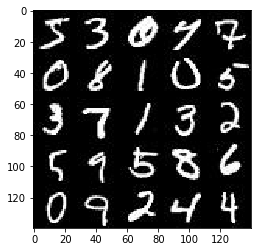### CelebA¶

The CelebFaces Attributes Dataset (CelebA) dataset contains over 200,000 celebrity images with annotations. Since you're going to be generating faces, you won't need the annotations. You can view the first number of examples by changing show_n_images.

In :
show_n_images = 25

"""
DON'T MODIFY ANYTHING IN THIS CELL
"""
mnist_images = helper.get_batch(glob(os.path.join(data_dir, 'img_align_celeba/*.jpg'))[:show_n_images], 28, 28, 'RGB')
pyplot.imshow(helper.images_square_grid(mnist_images, 'RGB'))

Out:
<matplotlib.image.AxesImage at 0x7761860>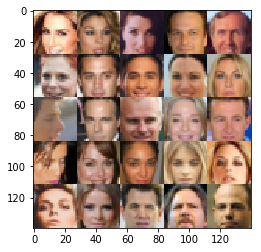## Preprocess the Data¶

Since the project's main focus is on building the GANs, we'll preprocess the data for you. The values of the MNIST and CelebA dataset will be in the range of -0.5 to 0.5 of 28x28 dimensional images. The CelebA images will be cropped to remove parts of the image that don't include a face, then resized down to 28x28.

The MNIST images are black and white images with a single color channel while the CelebA images have 3 color channels (RGB color channel).

## Build the Neural Network¶

You'll build the components necessary to build a GANs by implementing the following functions below:

• model_inputs
• discriminator
• generator
• model_loss
• model_opt
• train

This will check to make sure you have the correct version of TensorFlow and access to a GPU

In :
"""
DON'T MODIFY ANYTHING IN THIS CELL
"""
from distutils.version import LooseVersion
import warnings
import tensorflow as tf

# Check TensorFlow Version
assert LooseVersion(tf.__version__) >= LooseVersion('1.0'), 'Please use TensorFlow version 1.0 or newer.  You are using {}'.format(tf.__version__)
print('TensorFlow Version: {}'.format(tf.__version__))

# Check for a GPU
if not tf.test.gpu_device_name():
warnings.warn('No GPU found. Please use a GPU to train your neural network.')
else:
print('Default GPU Device: {}'.format(tf.test.gpu_device_name()))

TensorFlow Version: 1.1.0
Default GPU Device: /gpu:0


### Input¶

Implement the model_inputs function to create TF Placeholders for the Neural Network. It should create the following placeholders:

• Real input images placeholder with rank 4 using image_width, image_height, and image_channels.
• Z input placeholder with rank 2 using z_dim.
• Learning rate placeholder with rank 0.

Return the placeholders in the following the tuple (tensor of real input images, tensor of z data)

In :
import problem_unittests as tests

def model_inputs(image_width, image_height, image_channels, z_dim):
"""
Create the model inputs
:param image_width: The input image width
:param image_height: The input image height
:param image_channels: The number of image channels
:param z_dim: The dimension of Z
:return: Tuple of (tensor of real input images, tensor of z data, learning rate)
"""
# TODO: Implement Function
inputs_real = tf.placeholder(tf.float32, (None, image_width, image_height, image_channels), name='input_real')
inputs_z = tf.placeholder(tf.float32, (None, z_dim), name='input_z')
learning_rate = tf.placeholder(tf.float32, (None))
return inputs_real, inputs_z, learning_rate

"""
DON'T MODIFY ANYTHING IN THIS CELL THAT IS BELOW THIS LINE
"""
tests.test_model_inputs(model_inputs)

Tests Passed


### Discriminator¶

Implement discriminator to create a discriminator neural network that discriminates on images. This function should be able to reuse the variabes in the neural network. Use tf.variable_scope with a scope name of "discriminator" to allow the variables to be reused. The function should return a tuple of (tensor output of the discriminator, tensor logits of the discriminator).

In :
def discriminator(images, reuse=False):
"""
Create the discriminator network
:param image: Tensor of input image(s)
:param reuse: Boolean if the weights should be reused
:return: Tuple of (tensor output of the discriminator, tensor logits of the discriminator)
"""
# TODO: Implement Function
alpha=0.2
x = images
with tf.variable_scope('discriminator', reuse=reuse):
x = tf.layers.conv2d(x, 64, 4, strides=2, padding="same")
x = tf.layers.batch_normalization(x, training=True)
x = tf.maximum(alpha * x, x)
#x = tf.layers.dropout(x, 0.5)

x = tf.layers.conv2d(x, 128, 4, strides=2, padding="same")
x = tf.layers.batch_normalization(x, training=True)
x = tf.maximum(alpha * x, x)
#x = tf.layers.dropout(x, 0.5)

x = tf.layers.conv2d(x, 256, 4, strides=2, padding="same")
x = tf.layers.batch_normalization(x, training=True)
x = tf.maximum(alpha * x, x)
#x = tf.layers.dropout(x, 0.5)

x = tf.reshape(x, (-1, 4*4*256))
logits = tf.layers.dense(x, 1)
out = tf.sigmoid(logits)

return out, logits

# The following is a dense layer only model
#image_shape = images.shape
#image_shape_flatted = image_shape*image_shape*image_shape

#n_units=128*2
#alpha=0.01
#with tf.variable_scope('discriminator', reuse=reuse):
#images = tf.contrib.layers.flatten(images)
# Hidden layer
#print(images.shape)
#h1 = tf.layers.dense(images, n_units, activation=None)
# Leaky ReLU
#h1 = tf.maximum(alpha * h1, h1)
#print(h1.shape)
#h1 = tf.reshape(h1, [-1, image_shape_flatted])

#logits = tf.layers.dense(h1, 1, activation=None)
#out = tf.sigmoid(logits)
#print(logits.shape)
#print(out.shape)

#return out, logits

"""
DON'T MODIFY ANYTHING IN THIS CELL THAT IS BELOW THIS LINE
"""
tests.test_discriminator(discriminator, tf)

Tests Passed


### Generator¶

Implement generator to generate an image using z. This function should be able to reuse the variabes in the neural network. Use tf.variable_scope with a scope name of "generator" to allow the variables to be reused. The function should return the generated 28 x 28 x out_channel_dim images.

In :
def generator(z, out_channel_dim, is_train=True):
"""
Create the generator network
:param z: Input z
:param out_channel_dim: The number of channels in the output image
:param is_train: Boolean if generator is being used for training
:return: The tensor output of the generator
"""
# TODO: Implement Function
reuse = not is_train
alpha=0.2
with tf.variable_scope('generator', reuse=reuse):
x = tf.layers.dense(z, 4*4*512)

x = tf.reshape(x, (-1,4,4,512))
x = tf.layers.batch_normalization(x,training=is_train)
#x = tf.layers.dropout(x, 0.5)
x = tf.maximum(alpha * x, x)
#print(x.shape)
x = tf.layers.conv2d_transpose(x, 256, 4, strides=1, padding="valid")
x = tf.layers.batch_normalization(x,training=is_train)
x = tf.maximum(alpha * x, x)
#print(x.shape)
x = tf.layers.conv2d_transpose(x, 128, 4, strides=2, padding="same")
x = tf.layers.batch_normalization(x,training=is_train)
x = tf.maximum(alpha * x, x)
#print(x.shape)
x = tf.layers.conv2d_transpose(x, out_channel_dim, 4, strides=2, padding="same")
#x = tf.maximum(alpha * x, x)

logits = x
out = tf.tanh(logits)
#print(logits.shape)
#print(out.shape)

return out

# The following is a dense layer only model
#reuse = not is_train
#n_units=128*2
#alpha=0.01
#with tf.variable_scope('generator', reuse=reuse):
#print(z.shape)
#z = tf.contrib.layers.flatten(z)
#print(z.shape)
# Hidden layer
#h1 = tf.layers.dense(z, n_units, activation=None)
# Leaky ReLU
#h1 = tf.maximum(alpha * h1, h1)

# Logits and tanh output
#logits = tf.layers.dense(h1, 28*28*out_channel_dim, activation=None)
#out = tf.tanh(logits)
#print(out.shape)
#out = tf.reshape(out, [-1,28,28,out_channel_dim])
#print(out.shape)

#return out

"""
DON'T MODIFY ANYTHING IN THIS CELL THAT IS BELOW THIS LINE
"""
tests.test_generator(generator, tf)

Tests Passed


### Loss¶

Implement model_loss to build the GANs for training and calculate the loss. The function should return a tuple of (discriminator loss, generator loss). Use the following functions you implemented:

• discriminator(images, reuse=False)
• generator(z, out_channel_dim, is_train=True)
In :
def model_loss(input_real, input_z, out_channel_dim):
"""
Get the loss for the discriminator and generator
:param input_real: Images from the real dataset
:param input_z: Z input
:param out_channel_dim: The number of channels in the output image
:return: A tuple of (discriminator loss, generator loss)
"""
# TODO: Implement Function
smooth = 0.1
_, d_logits_real = discriminator(input_real, reuse=False)
fake = generator(input_z, out_channel_dim, is_train=True)
d_logits_fake = discriminator(fake, reuse=True)
#print(input_real)
#print(input_z)
#print(d_logits_real.shape)
#print(d_logits_fake)
# Calculate losses
d_loss_real = tf.reduce_mean(
tf.nn.sigmoid_cross_entropy_with_logits(logits=d_logits_real,
labels=tf.ones_like(d_logits_real) * (1 - smooth)))
d_loss_fake = tf.reduce_mean(
tf.nn.sigmoid_cross_entropy_with_logits(logits=d_logits_fake,
labels=tf.zeros_like(d_logits_fake)))
d_loss = d_loss_real + d_loss_fake

g_loss = tf.reduce_mean(
tf.nn.sigmoid_cross_entropy_with_logits(logits=d_logits_fake,
labels=tf.ones_like(d_logits_fake)))
return d_loss, g_loss

"""
DON'T MODIFY ANYTHING IN THIS CELL THAT IS BELOW THIS LINE
"""
tests.test_model_loss(model_loss)

Tests Passed


### Optimization¶

Implement model_opt to create the optimization operations for the GANs. Use tf.trainable_variables to get all the trainable variables. Filter the variables with names that are in the discriminator and generator scope names. The function should return a tuple of (discriminator training operation, generator training operation).

In :
def model_opt(d_loss, g_loss, learning_rate, beta1):
"""
Get optimization operations
:param d_loss: Discriminator loss Tensor
:param g_loss: Generator loss Tensor
:param learning_rate: Learning Rate Placeholder
:param beta1: The exponential decay rate for the 1st moment in the optimizer
:return: A tuple of (discriminator training operation, generator training operation)
"""
# TODO: Implement Function
# Optimizers

# Get the trainable_variables, split into G and D parts
t_vars = tf.trainable_variables()
g_vars = [var for var in t_vars if var.name.startswith('generator')]
d_vars = [var for var in t_vars if var.name.startswith('discriminator')]
all_update_ops = tf.get_collection(tf.GraphKeys.UPDATE_OPS)

g_update_ops = [var for var in all_update_ops if var.name.startswith('generator')]
d_update_ops = [var for var in all_update_ops if var.name.startswith('discriminator')]

with tf.control_dependencies(d_update_ops):
with tf.control_dependencies(g_update_ops):
return d_train_opt, g_train_opt

"""
DON'T MODIFY ANYTHING IN THIS CELL THAT IS BELOW THIS LINE
"""
tests.test_model_opt(model_opt, tf)

Tests Passed


## Neural Network Training¶

### Show Output¶

Use this function to show the current output of the generator during training. It will help you determine how well the GANs is training.

In :
"""
DON'T MODIFY ANYTHING IN THIS CELL
"""
import numpy as np

def show_generator_output(sess, n_images, input_z, out_channel_dim, image_mode):
"""
Show example output for the generator
:param sess: TensorFlow session
:param n_images: Number of Images to display
:param input_z: Input Z Tensor
:param out_channel_dim: The number of channels in the output image
:param image_mode: The mode to use for images ("RGB" or "L")
"""
cmap = None if image_mode == 'RGB' else 'gray'
z_dim = input_z.get_shape().as_list()[-1]
example_z = np.random.uniform(-1, 1, size=[n_images, z_dim])

samples = sess.run(
generator(input_z, out_channel_dim, False),
feed_dict={input_z: example_z})

images_grid = helper.images_square_grid(samples, image_mode)
pyplot.imshow(images_grid, cmap=cmap)
pyplot.show()


### Train¶

Implement train to build and train the GANs. Use the following functions you implemented:

• model_inputs(image_width, image_height, image_channels, z_dim)
• model_loss(input_real, input_z, out_channel_dim)
• model_opt(d_loss, g_loss, learning_rate, beta1)

Use the show_generator_output to show generator output while you train. Running show_generator_output for every batch will drastically increase training time and increase the size of the notebook. It's recommended to print the generator output every 100 batches.

In :
import time
def train(epoch_count, batch_size, z_dim, learning_rate, beta1, get_batches, data_shape, data_image_mode):
"""
Train the GAN
:param epoch_count: Number of epochs
:param batch_size: Batch Size
:param z_dim: Z dimension
:param learning_rate: Learning Rate
:param beta1: The exponential decay rate for the 1st moment in the optimizer
:param get_batches: Function to get batches
:param data_shape: Shape of the data
:param data_image_mode: The image mode to use for images ("RGB" or "L")
"""
# TODO: Build Model
inputs_real, inputs_z, lr = model_inputs(data_shape, data_shape, data_shape, z_dim)
d_loss, g_loss = model_loss(inputs_real, inputs_z, data_shape[-1])
d_train_opt, g_train_opt = model_opt(d_loss, g_loss, learning_rate, beta1)
step = 0

with tf.Session() as sess:
sess.run(tf.global_variables_initializer())
for epoch_i in range(epoch_count):
for batch_images in get_batches(batch_size):
# TODO: Train Model
start_time = time.time()
step = step+1
batch_images = batch_images * 2
# Sample random noise for G
batch_z = np.random.uniform(-1, 1, size=(batch_size, z_dim))
#print('batch_z shape=',batch_z.shape)
# Run optimizers
_ = sess.run(d_train_opt, feed_dict={inputs_real: batch_images, inputs_z: batch_z, lr:learning_rate})
_ = sess.run(g_train_opt, feed_dict={inputs_z: batch_z, lr:learning_rate})

if step % 100 == 0:
train_loss_d = d_loss.eval({inputs_z:batch_z, inputs_real: batch_images})
train_loss_g = g_loss.eval({inputs_z:batch_z})
print("Epoch {}/{} Step {}...".format(epoch_i+1, epoch_count, step),
"Discriminator Loss: {:.4f}...".format(train_loss_d),
"Generator Loss: {:.4f}".format(train_loss_g),
"... Time spent={:.4f}".format(time.time() - start_time))

if step % 200 == 0:
show_generator_output(sess, 25, inputs_z, data_shape, data_image_mode)



### MNIST¶

Test your GANs architecture on MNIST. After 2 epochs, the GANs should be able to generate images that look like handwritten digits. Make sure the loss of the generator is lower than the loss of the discriminator or close to 0.

In :
batch_size = 100
z_dim = 100
learning_rate = 0.0001
beta1 = 0.2

"""
DON'T MODIFY ANYTHING IN THIS CELL THAT IS BELOW THIS LINE
"""
epochs = 2

mnist_dataset = helper.Dataset('mnist', glob(os.path.join(data_dir, 'mnist/*.jpg')))
with tf.Graph().as_default():
train(epochs, batch_size, z_dim, learning_rate, beta1, mnist_dataset.get_batches,
mnist_dataset.shape, mnist_dataset.image_mode)

Epoch 1/2 Step 100... Discriminator Loss: 1.3952... Generator Loss: 0.5184 ... Time spent=0.1760
Epoch 1/2 Step 200... Discriminator Loss: 1.1850... Generator Loss: 0.6578 ... Time spent=0.0880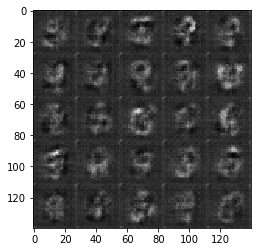Epoch 1/2 Step 300... Discriminator Loss: 1.1668... Generator Loss: 0.6345 ... Time spent=0.0890
Epoch 1/2 Step 400... Discriminator Loss: 1.3749... Generator Loss: 0.4750 ... Time spent=0.0870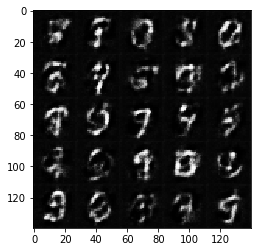Epoch 1/2 Step 500... Discriminator Loss: 1.1364... Generator Loss: 1.3730 ... Time spent=0.0900
Epoch 1/2 Step 600... Discriminator Loss: 1.1792... Generator Loss: 0.6140 ... Time spent=0.0880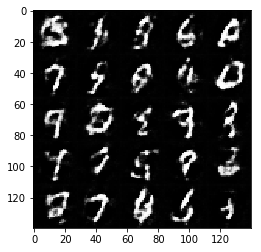Epoch 2/2 Step 700... Discriminator Loss: 1.0652... Generator Loss: 0.8248 ... Time spent=0.0880
Epoch 2/2 Step 800... Discriminator Loss: 1.6900... Generator Loss: 0.3242 ... Time spent=0.0910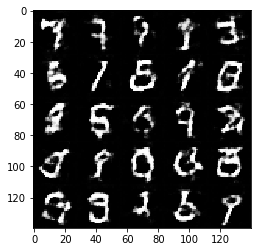Epoch 2/2 Step 900... Discriminator Loss: 1.2951... Generator Loss: 0.5190 ... Time spent=0.0890
Epoch 2/2 Step 1000... Discriminator Loss: 1.6801... Generator Loss: 0.3297 ... Time spent=0.0900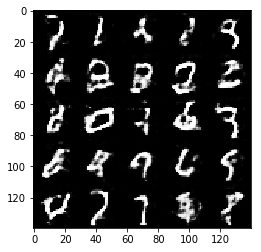Epoch 2/2 Step 1100... Discriminator Loss: 1.2643... Generator Loss: 1.0945 ... Time spent=0.0920
Epoch 2/2 Step 1200... Discriminator Loss: 1.1050... Generator Loss: 0.9563 ... Time spent=0.0880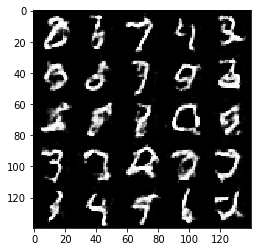### CelebA¶

Run your GANs on CelebA. It will take around 20 minutes on the average GPU to run one epoch. You can run the whole epoch or stop when it starts to generate realistic faces.

In :
batch_size = 100
z_dim = 100
learning_rate = 0.001
beta1 = 0.5

"""
DON'T MODIFY ANYTHING IN THIS CELL THAT IS BELOW THIS LINE
"""
epochs = 10

celeba_dataset = helper.Dataset('celeba', glob(os.path.join(data_dir, 'img_align_celeba/*.jpg')))
with tf.Graph().as_default():
train(epochs, batch_size, z_dim, learning_rate, beta1, celeba_dataset.get_batches,
celeba_dataset.shape, celeba_dataset.image_mode)

Epoch 1/10 Step 100... Discriminator Loss: 0.9593... Generator Loss: 1.3587 ... Time spent=0.1910
Epoch 1/10 Step 200... Discriminator Loss: 2.1685... Generator Loss: 0.2420 ... Time spent=0.0890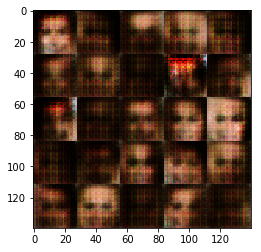Epoch 1/10 Step 300... Discriminator Loss: 1.2852... Generator Loss: 0.9545 ... Time spent=0.0880
Epoch 1/10 Step 400... Discriminator Loss: 1.4207... Generator Loss: 0.9330 ... Time spent=0.0860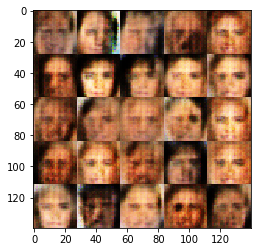Epoch 1/10 Step 500... Discriminator Loss: 1.3461... Generator Loss: 0.7174 ... Time spent=0.0880
Epoch 1/10 Step 600... Discriminator Loss: 1.3332... Generator Loss: 0.7420 ... Time spent=0.0870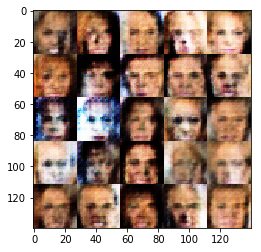Epoch 1/10 Step 700... Discriminator Loss: 1.7075... Generator Loss: 0.3425 ... Time spent=0.0880
Epoch 1/10 Step 800... Discriminator Loss: 1.3035... Generator Loss: 0.6241 ... Time spent=0.0870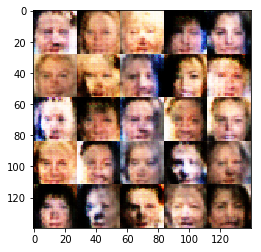Epoch 1/10 Step 900... Discriminator Loss: 1.3626... Generator Loss: 0.5287 ... Time spent=0.0840
Epoch 1/10 Step 1000... Discriminator Loss: 1.4340... Generator Loss: 0.6404 ... Time spent=0.0900